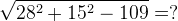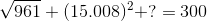# Square Root & Cube Root - Quantitative Aptitude Questions with Answers

Questions
1 Calculate the appropriate value to replace the question mark(?)A 31
B 24
C 23
D 21

2 Calculate the appropriate value to replace the question mark(?)A 26
B 29
C 30
D 31

3 Calculate the appropriate value to replace the question mark(?)A 43.882295
B 43.759936
C 44.789451
D 44.478794

4 Calculate the appropriate value to replace the question mark(?)A 194
B 196
C 198
D 200

5 Calculate the appropriate value to replace the question mark(?)A 45
B 50
C 55
D 60